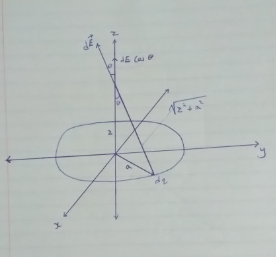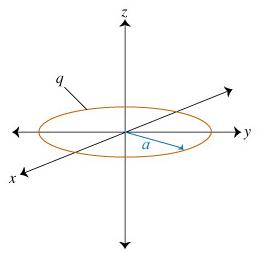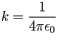# Problem: Consider a uniformly charged ring in the xy plane, centered at the origin. The ring has radius α and positive charge q distributed evenly along its circumference.1. What is the magnitude of the electric field along the positive z axis?Use k in your answer, where .   ( E(z)=? )

###### FREE Expert Solution

Electric field:

$\overline{){\mathbf{E}}{\mathbf{=}}\frac{\mathbf{k}\mathbf{q}}{{\mathbf{r}}^{\mathbf{2}}}}$cos θ = z/sqrt(z2+a2)

dE(z) =  dE cos θ

From the boxed equation:

E = kq/[sqrt(z2 + a2)]2 = kq/sqrt(z2 + a2)

83% (473 ratings)###### Problem DetailsConsider a uniformly charged ring in the xy plane, centered at the origin. The ring has radius α and positive charge q distributed evenly along its circumference.

1. What is the magnitude of the electric field along the positive z axis?

Use k in your answer, where.   ( E(z)=? )